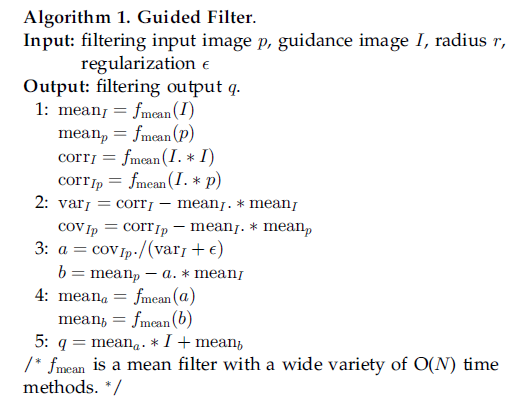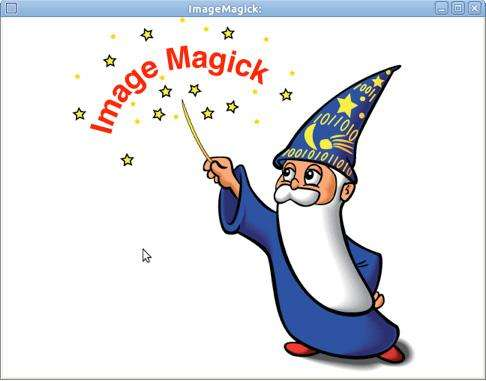2018-12-17 09:10:00 weixin_30847939 阅读数 12
• ###### MATLAB图像处理

全面系统的学习MATLAB在图像处理中的应用

20184 人正在学习 去看看 魏伟
• 图像处理的几种方法 1.使用skimage
name details name details name details
astronaut 宇航员图片 coffee 一杯咖啡图片 lena lena图片
camera 拿相机的人图片 coins 硬币 moon 月亮
checkerboard 棋盘 horse page 一页书
chelsea 小猫图片 hubble_deep_field 星空 text 文字
clock 时钟 immunohistochemistry 结肠
``````import numpy as np
import scipy
import matplotlib.pyplot as plt
from skimage import io,data
img=data.chelsea()
#读取图片
#图片变换
[m,n,l]=img.shape
img1=skimage.transform.resize(img,(int(m*0.6),int(n*0.6),l),mode='reflect')
#保存图片
io.imsave('d:/python/loli1.jpg',img1)
io.imshow(img1)
plt.show()
# 显示图片信息
print (type(img))  #显示类型
print (img.shape)  #显示尺寸
print (img.shape)  #图片宽度
print (img.shape)  #图片高度
print (img.shape)  #图片通道数
print (img.size)   #显示总像素个数
print (img.max())  #最大像素值
print (img.min())  #最小像素值
print (img.mean()) #像素平均值``````
``````C:\ProgramData\Anaconda2\lib\site-packages\skimage\util\dtype.py:122: UserWarning: Possible precision loss when converting from float64 to uint8
.format(dtypeobj_in, dtypeobj_out))
````````````<type 'numpy.ndarray'>
(415L, 518L, 3L)
415
518
3
644910
255
0
111.793140128
``````
2 使用scipy.misc(miscellaneous routines)不推荐,很多已经deprecated

Various utilities that don’t have another home.
Note that Pillow (https://python-pillow.org/) is not a dependency of SciPy, but the image manipulation functions indicated in the list below are not available without it.

Deprecated functions:

col 1 col 2
bytescale(*args, **kwds) bytescale is deprecated!
fromimage(*args, **kwds) fromimage is deprecated!
imfilter(*args, **kwds) imfilter is deprecated!
imresize(*args, **kwds) imresize is deprecated!
imrotate(*args, **kwds) imrotate is deprecated!
imsave(*args, **kwds) imsave is deprecated!
imshow(*args, **kwds) imshow is deprecated!
toimage(*args, **kwds) toimage is deprecated!
``````from scipy import misc
import matplotlib.pyplot as plt
import numpy as np
# 读取图片
# 改变大小
img1=scipy.misc.imresize(img,(111,80),interp='nearest')
# 显示图片
#misc.imshow(img) have been deprecated
plt.imshow(img)
plt.show()
misc.imsave('d:/python/loli1.jpg',img)``````3 使用PIL做图像处理

3.1 Reading and Writing Images : open( infilename ) , save( outfilename )

3.2 Cutting and Pasting and Merging Images :

crop() : 从图像中提取出某个矩形大小的图像。它接收一个四元素的元组作为参数,各元素为(left, upper, right, lower),坐标系统的原点(0, 0)是左上角。
paste() :
merge()

3.3 几何变换

`````` box = (100, 100, 200, 200)
region = im.crop(box)
region.show()
region = region.transpose(Image.ROTATE_180)
region.show()
im.paste(region, box)
im.show()``````
``````#旋转一幅图片
def roll(image, delta):
"Roll an image sideways"

xsize, ysize = image.size

delta = delta % xsize
if delta == 0: return image

part1 = image.crop((0, 0, delta, ysize))
part2 = image.crop((delta, 0, xsize, ysize))
image.paste(part2, (0, 0, xsize-delta, ysize))
image.paste(part1, (xsize-delta, 0, xsize, ysize))

return image``````
``````#简单的几何变换
out = im.resize((128, 128))                     #
out = im.rotate(45)                             #逆时针旋转 45 度角。
out = im.transpose(Image.FLIP_LEFT_RIGHT)       #左右对换。
out = im.transpose(Image.FLIP_TOP_BOTTOM)       #上下对换。
out = im.transpose(Image.ROTATE_90)             #旋转 90 度角。
out = im.transpose(Image.ROTATE_180)            #旋转 180 度角。
out = im.transpose(Image.ROTATE_270)            #旋转 270 度角。``````
``````from PIL import Image
im=Image.open('d:/python/loli.jpg')
print im.format,im.size,im.mode
im.show()``````
``````JPEG (518, 415) RGB
``````
``````box = (100, 100, 200, 200)
region = im.crop(box)
region.show()
region = region.transpose(Image.ROTATE_180)
region.show()
im.paste(region, box)
im.show()``````
``````out = im.resize((128, 128))                     #
out = im.rotate(45)                             #逆时针旋转 45 度角。
out = im.transpose(Image.FLIP_LEFT_RIGHT)       #左右对换。
out = im.transpose(Image.FLIP_TOP_BOTTOM)       #上下对换。
out = im.transpose(Image.ROTATE_90)             #旋转 90 度角。
out = im.transpose(Image.ROTATE_180)            #旋转 180 度角。
out = im.transpose(Image.ROTATE_270)
out.show()``````
• 以上是图像处理的几种方法的内容，更多 图像处理 python 的内容，请您使用右上方搜索功能获取相关信息。

2012-03-21 17:09:52 smells2 阅读数 9689
• ###### MATLAB图像处理

全面系统的学习MATLAB在图像处理中的应用

20184 人正在学习 去看看 魏伟

0.知识储备

```typedef struct tagBITMAPFILEHEADER {
WORD    bfType;
DWORD   bfSize;
WORD    bfReserved1;
WORD    bfReserved2;
DWORD   bfOffBits;

```typedef struct tagBITMAPINFOHEADER{
DWORD      biSize;
LONG       biWidth;
LONG       biHeight;
WORD       biPlanes;
WORD       biBitCount;
DWORD      biCompression;
DWORD      biSizeImage;
LONG       biXPelsPerMeter;
LONG       biYPelsPerMeter;
DWORD      biClrUsed;
DWORD      biClrImportant;

1.将彩色图像转换为灰度图像

```/********************************************
*	   将文件转换成灰度图像		    *
********************************************/
int CBitMapFile::turn2Gray()
{
if (m_imageArray == NULL)
{
return -2;
}

for (int i =0,j= 0,k=0;i<m_sizeImage;)
{
//转换为灰度图像，注意填充的字节
BYTE blue,green,red,gray;

blue = m_imageArray[i];
green = m_imageArray[i+1];
red = m_imageArray[i+2];

m_realColorImageArray[k] = blue;
m_realColorImageArray[k+1] = green;
m_realColorImageArray[k+2] = red;

gray = (BYTE)(0.3*red+0.59*green+0.11*blue);

m_imageArray[i] = gray;
m_imageArray[i+1] = gray;
m_imageArray[i+2] = gray;

m_realImageArray[j] = m_imageArray[i];

i += 3;
k += 3;
j++;

////跳过填充字节
if (j % m_width == 0)
{
}

}

return 1;
}```

2.图像转换为ASNII码文件

```/******************************************
*对图像的每个像素做文本映射，最终写入文件*
******************************************/
int CBitMapFile::turn2Txt(CFile& txtFile)
{
char* _txtBuf = new char[m_width*m_height+2*m_height];
memset(_txtBuf,0,m_width*m_height+2*m_height);

//文本映射
char txtMap = {'@','\$','#','%','!','~','^','`'};

char* _buf = new char[m_width+2];
memset(_buf,0,m_width+2);
//TRACE(_T("\'\\r\'=%x,\'\\n\'=%x"),'\r','\n');
for (int i = m_height-1;i>=0;i--)
{
for (int j = 0;j<m_width;j++)
{
_buf[j] = txtMap[m_realImageArray[i*m_width+j]>>5];

}

_buf[m_width] = '\r';
_buf[m_width+1] = '\n';

for (int k=0;k<m_width+2;k++)
{
_txtBuf[(m_height-1-i)*m_width+k+(m_height-1-i)*2] = _buf[k];

}
}

txtFile.Write(_txtBuf,sizeof(char)*(m_width*m_height+2*m_height));

delete _txtBuf;
delete _buf;
return 1;
}```3.直方图均衡化```/********************************************
*	对bmp文件的每个像素的灰度值进行统计		*
********************************************/
{
for (int i = 0;i<m_width*m_height;i++)
{
m_grayStatistic[m_realImageArray[i]] += 1;

}
for (int i = 0;i<256;i++)
{
if (m_grayStatistic[i]>m_max)
{
m_max = m_grayStatistic[i];
}

}
//TRACE(_T("m_max = %d\n"),m_max);
return 1;
}
/********************************************
*	对bmp文件的每个像素的灰度值进行归一化	*
*		并计算每个像素灰度值的概率			*
********************************************/
int CBitMapFile::turn2One()
{
for (int i =0;i<256;i++)
{
m_grayTurn2OneData[i] = (double)m_grayStatistic[i]/m_max;
m_grayFrequencyPerLevel[i] = (double)m_grayStatistic[i]/(m_width*m_height);

}
m_maxFrequency = m_max/(m_width*m_height);
return 1;
}

/********************************************
*	清除统计数组、归一化数组、频率数组		*
********************************************/
void CBitMapFile::clearup()
{
memset(m_grayStatistic,0,sizeof(LONG)*256);
memset(m_grayTurn2OneData,0,sizeof(double)*256);
memset(m_grayFrequencyPerLevel,0,sizeof(double)*256);
m_max = 0;
m_maxFrequency = 0.0f;
}
/********************************************
*				灰度均衡化					*
********************************************/
void CBitMapFile::equation(CFile& file)
{
double _temp =0.0f;
double* _array = new double;
memset(_array,0,sizeof(double)*256);
int* _intArray = new int;
memset(_intArray,0,sizeof(int)*256);
BYTE* _writeArray = new BYTE[m_sizeImage];
memset(_writeArray,0,sizeof(BYTE)*m_sizeImage);
for(int i = 0;i<256;i++)
{
_array[i] = ((m_grayFrequencyPerLevel[i])*255)+_temp;
_temp = _array[i];

}
for (int i = 0;i<256;i++)
{
_intArray[i] = (int)(_array[i]);
}
for (int i = 0,j = 0;i<m_sizeImage;)
{

_writeArray[i] = _intArray[m_realImageArray[j]];
_writeArray[i+1] = _intArray[m_realImageArray[j]];
_writeArray[i+2] = _intArray[m_realImageArray[j]];
j++;
i += 3;
if (j%m_width == 0)
{
{
_writeArray[i+k] = 0;

}
}
}
file.Write(_writeArray,sizeof(BYTE)*m_sizeImage);
delete _array;
delete _intArray;
delete _writeArray;
}```4.伽马校正

```/********************************************
*				伽马校正					*
********************************************/
void CBitMapFile::gammaCorrection(double gamma,CFile& file)
{
//数据准备
double* _correctData = new double[m_width*m_height];
BYTE* _writeArray = new BYTE[m_sizeImage];
memset(_writeArray,0,sizeof(BYTE)*m_sizeImage);
memset(_correctData,0,sizeof(double)*m_width*m_height);
//算法
for (int i = 0;i<m_width*m_height;i++)
{
_correctData[i] = (double)m_realImageArray[i]/255;

_correctData[i] = pow(_correctData[i],gamma)*255;

}
//写入文件准备
for (int i = 0,j = 0;i<m_sizeImage;)
{
_writeArray[i] = (BYTE)_correctData[j];
_writeArray[i+1] = (BYTE)_correctData[j];
_writeArray[i+2] = (BYTE)_correctData[j];
j++;
i += 3;
if (j%m_width == 0)
{
{
_writeArray[i+k] = 0;

}
}
}
//写入文件
file.Write(_writeArray,sizeof(BYTE)*m_sizeImage);

delete _writeArray;
delete _correctData;
}```4.哈尔小波变换（HWT，haar wavelet tansform）

Matrix.h

```#pragma once
#define ZERO 0	//零阵
#define IDENTITY 1//单位阵
#define HAAR 2//HAAR小波矩阵
#define UNSQUARE -1//非方阵
#define UNSAME -2//非同型矩阵
#define UNSAME -2//非同型矩阵
#define UNEVEN -3//行列非偶数

class CMatrix
{
public:
CMatrix(int r,int c);
CMatrix(CMatrix& m);
CMatrix(double* d,int r,int c);
CMatrix(BYTE* d,int r,int c);
~CMatrix();
int inital(int type);//初始化为单位阵，或特殊矩阵（以后扩展）
#ifdef _DEBUG
void display();
#endif
//////////////////////////////////////////////////////////////////////////
public:
CMatrix& transpose();//矩阵的转置
void nonZero();
//////////////////////////////////////////////////////////////////////////
public:
CMatrix& operator=(CMatrix& m1);
friend CMatrix operator+(CMatrix& m1,CMatrix& m2);
friend CMatrix operator-(CMatrix& m1,CMatrix& m2);
friend CMatrix operator*(CMatrix& m1,double& a);
friend CMatrix operator*(CMatrix& m1,CMatrix& m2);
//////////////////////////////////////////////////////////////////////////
public:
inline int getRow(){return m_row;}
inline int getCol(){return m_col;}
//inline CString getName(){return m_name;}
inline double getElem(int r,int c){return m_data[r*m_row+c];}
inline double* getData(){return m_data;}
//////////////////////////////////////////////////////////////////////////
protected:
int m_row;
int m_col;
double* m_data;
//	CString m_name;
};
```

Matrix.cpp

```#include "StdAfx.h"

#include "MatrixBase.h"
#ifdef _DEBUG
#include <iostream>
#endif

CMatrix::CMatrix(int r,int c)
{
m_row = r;
m_col = c;
m_data = new double[r*c];
memset(m_data,0,sizeof(double)*r*c);
}
CMatrix::CMatrix(double* d,int r,int c)
{
m_row = r;
m_col = c;
m_data = new double[r*c];
for (int i = 0;i<r*c;i++)
{
m_data[i] = d[i];
}
}
CMatrix::CMatrix(BYTE* d,int r,int c)
{
m_row = r;
m_col = c;
m_data = new double[r*c];
for (int i = 0;i<r*c;i++)
{
m_data[i] = (double)d[i];
}
}
CMatrix::CMatrix(CMatrix& m)
{
this->m_row = m.m_row;
this->m_col = m.m_col;
m_data = new double[m_row*m_col];
for (int i = 0;i<m_row*m_col;i++)
{
this->m_data[i] = m.m_data[i];
TRACE(_T("data[%d]=%f\n"),i,this->m_data[i]);
}
}
CMatrix::~CMatrix()
{
delete[] m_data;
}
int CMatrix::inital(int type)
{

switch(type)
{
case IDENTITY:
if (m_row != m_col)
{
return UNSQUARE;
}
for (int i=0;i<m_col;i++)
{
m_data[i*m_row+i] = 1;
}
break;
case HAAR:
if (m_row != m_col)
{
return UNSQUARE;
}
if (m_row%2 != 0)
{
return UNEVEN;
}
for(int i = 0;i<m_row/2;i++)
{
m_data[i*m_row+i*2] = 1;
m_data[i*m_row+i*2+1] = 1;
}
for (int i = m_row/2,j = 0;i<m_row;i++,j++)
{
m_data[i*m_row+j*2] = -1;
m_data[i*m_row+j*2+1] = 1;
}
break;
default:
break;
}
return type;
}
CMatrix& CMatrix::operator=(CMatrix& m1)
{
ASSERT(m_row == m1.getRow() && m_col == m1.getCol());
for (int i = 0;i<m_row*m_col;i++)
{
m_data[i] = m1.getElem(i/m_row,i%m_row);
TRACE(_T("\'=\'data[%d]=%f\n"),i,m_data[i]);
}
return *this;
}
CMatrix operator+(CMatrix& m1,CMatrix& m2)
{
ASSERT(m1.m_row == m2.m_row && m1.m_col==m2.m_col);
CMatrix ret(m1.m_row,m1.m_col);
for (int i = 0;i<m1.m_row*m1.m_col;i++)
{
ret.m_data[i] = m1.m_data[i]+m2.m_data[i];
TRACE(_T("ret[%d]=%f\n"),i,ret.m_data[i]);
}
return ret;

}
CMatrix operator-(CMatrix& m1,CMatrix& m2)
{
ASSERT(m1.m_row == m2.m_row && m1.m_col==m2.m_col);
CMatrix ret(m1.m_row,m1.m_col);
for (int i = 0;i<m1.m_row*m1.m_col;i++)
{
ret.m_data[i] = m1.m_data[i]-m2.m_data[i];
TRACE(_T("ret[%d]=%f\n"),i,ret.m_data[i]);
}
return ret;
}
CMatrix operator*(CMatrix& m1,double& a)
{
CMatrix ret(m1);
for (int i = 0;i<m1.m_row*m1.m_col;i++)
{
ret.m_data[i] *=a;
TRACE(_T("ret[%d]=%f\n"),i,ret.m_data[i]);
}
return ret;
}
CMatrix operator*(CMatrix& m1,CMatrix& m2)
{
ASSERT(m1.m_col == m2.m_row);
CMatrix ret(m1.m_row,m2.m_col);
for (int i= 0;i<m1.m_row;i++)
{
for (int j = 0;j<m2.m_col;j++)
{
double _temp = 0;
for (int k = 0;k<m1.m_col;k++)
{
_temp += m1.m_data[i*m1.m_row+k]*m2.m_data[k*m2.m_row+j];
}
ret.m_data[i*m1.m_row+j] = _temp;
}
}
return ret;
}
CMatrix& CMatrix::transpose()
{
double* _newData = new double[m_row*m_col];

for (int i = 0;i<m_row;i++)
{
for (int j=0;j<m_col;j++)
{
_newData[j*m_col+i] = m_data[i*m_row+j];
}
}
delete m_data;
m_data = _newData;

return *this;

}
void CMatrix::nonZero()
{
for (int i = 0;i<m_row;i++)
{
for (int j = 0;j<m_col;j++)
{
if(m_data[i*m_row+j]<0)
{
m_data[i*m_row+j]=0;
}
}
}
}
#ifdef _DEBUG
void CMatrix::display()
{
std::cout<<"[\n";
for (int i = 0;i<m_row*m_col;i++)
{
std::cout<<m_data[i]<<" ,";
if ((i+1)%m_row == 0)
{
std::cout<<std::endl;
}
}
std::cout<<"]"<<std::endl;
}
#endif
```

```/********************************************
*				HAAR小波变换				*
********************************************/
void CBitMapFile::haarTransform(CFile& file)
{
CMatrix imageMatrix(m_realImageArray,m_height,m_width);
CMatrix haarMatrix_r(m_height,m_height);
CMatrix haarMatrix_c(m_width,m_width);
#ifdef _DEBUG
imageMatrix.display();
#endif
haarMatrix_c.inital(HAAR);
haarMatrix_c = haarMatrix_c.transpose();
haarMatrix_r.inital(HAAR);
imageMatrix = haarMatrix_r*imageMatrix;
double _d = 0.25*sqrt(2.0);
imageMatrix = imageMatrix*_d;
imageMatrix = imageMatrix*haarMatrix_c;
imageMatrix = imageMatrix*_d;

imageMatrix.nonZero();
#ifdef _DEBUG
imageMatrix.display();
#endif

double* _correctData = imageMatrix.getData();
BYTE* _writeArray = new BYTE[m_sizeImage];
memset(_writeArray,0,sizeof(BYTE)*m_sizeImage);

for (int i = 0,j = 0;i<m_sizeImage;)
{
_writeArray[i] = (BYTE)_correctData[j];
_writeArray[i+1] = (BYTE)_correctData[j];
_writeArray[i+2] = (BYTE)_correctData[j];
j++;
i += 3;
if (j%m_width == 0)
{
{
_writeArray[i+k] = 0;

}
}
}
file.Write(_writeArray,sizeof(BYTE)*m_sizeImage);

delete _writeArray;
//delete _correctData;
}```2018-03-26 17:06:07 Julialove102123 阅读数 5317
• ###### MATLAB图像处理

全面系统的学习MATLAB在图像处理中的应用

20184 人正在学习 去看看 魏伟## 中值滤波## 最大最小值滤波## 双边滤波## 引导滤波```def guidedfilter(I,p,r,eps):
'''I:引导图图；
p:输入图（p=I）;
r :半径:
eps:regulation
f:为窗口半径为r的均值滤波器；
corr：相关；
var：方差；
cov：协方差
'''
height,width = I.reshape()
m_I = cv2.boxFilter(I,-1,(r,r))  #f_mean(I) 均值滤波blur和盒式滤波一样
m_p = cv2.boxFilter(p,-1,(r,r))  #f_mean(p)

m_II = cv2.boxFilter(I*I,-1,(r,r)) #f_mean(I.*I)
m_Ip = cv2.boxFilter(I * p, -1, (r, r))  #f_mean(I.*p)

var_I = m_II-m_I*m_I   #求方差：corr_I -mean_I.*mean_I
cov_Ip = m_Ip - m_I * m_p  #协方差： #cov_Ip-mean_I.*mean_p

a = cov_Ip/(var_I+eps)  #cov_Ip./(var_I+eps)
b = m_p-a*m_I   #mean_p -a.*mean_I
m_a = cv2.boxFilter(a,-1,(r,r))  #mean_a
m_b = cv2.boxFilter(b,-1,(r,r))  #mean_b
return m_a*I+m_b```

2019-06-28 09:58:26 AugustMe 阅读数 853
• ###### MATLAB图像处理

全面系统的学习MATLAB在图像处理中的应用

20184 人正在学习 去看看 魏伟

1、水平翻转

2、竖直翻转

3、随机翻转角度

4、随机水平平移

5、随机竖直平移

6、随机错切变换

7、随机放大

8、颜色抖动

9、rescale
rescale的作用是对图片的每个像素值均乘上这个放缩因子，这个操作在所有其它变换操作之前执行，在一些模型当中，直接输入原图的像素值可能会落入激活函数的饱和区，因此设置放缩因子为1/255，把像素值放缩到0和1之间有利于模型的收敛，避免神经元饱和。图片经过rescale之后，保存到本地的图片用肉眼看是没有任何区别的。

10、fill_mode
fill_mode为填充模式，如前面提到，当对图片进行平移、放缩、错切等操作时，图片中会出现一些缺失的地方，就由fill_mode中的参数确定。包括：“constant”、“nearest”（默认）、“reflect”和“wrap”。

## 通常来说我们不会只使用单一的数据增加方法，而是会多种方式结合使用

2018-05-02 22:31:27 fanyun_01 阅读数 17529
• ###### MATLAB图像处理

全面系统的学习MATLAB在图像处理中的应用

20184 人正在学习 去看看 魏伟

1.OpenCV，主要以算法形式，展示其实现；也就是说，它实际提供的是各种图像处理算法。若需具体应用，需要组合其算法以实现某个功能。OpenCV 的全称 Open Source Computer Vision Library，是一个基于BSD许可（开源）发行的跨平台计算机视觉库,又名 “开源计算机视觉库”。OpenCV 是一个开源发行的跨平台计算机视觉库，可运行在 Windows、Android、Maemo、FreeBSD、OpenBSD、iOS、Linux 和 Mac OS 等平台。使用者可在 SourceForge 获得官方版本, 或从 SVN 获得开发版本。OpenCV 也用 CMake。

OpenCV 轻量且高效 —— 由一系列 C 函数和少量 C++ 类构成，同时提供了 Python、Ruby、MATLAB 等语言接口，实现了图像处理和计算机视觉方面的很多通用算法。

OpenCV 用 C++ 语言编写，它的主要接口也是 C++ 语言，但依然保留了大量的 C 语言接口。OpenCV 库也有 Python、Java、MATLAB/OCTAVE (版本 2.5) 接口。这些语言的 API 接口函数，可通过在线文档获得。如今，OpenCV 也提供对于 C#、Ch、Ruby 的支持。

OpenCV 所有新开发和算法都采用 C++ 接口。

OpenCV 拥有包括 500 多个 C 函数的跨平台中、高层 API。OpenCV 不依赖于其它的外部库 —— 尽管也可使用某些外部库。

1999 年 Intel 开始建立 OpenCV，如今由 Willow Garage 提供支持。

OpenCV 为 Intel ® Integrated Performance Primitives (IPP) 提供了透明接口。这意味着，如果有为特定处理器优化的 IPP 库， OpenCV 将在运行时自动加载这些库。OpenCV 2.0 版代码已显著优化，无需 IPP 来提升性能，故 2.0 版不再提供 IPP 接口。

2010 年 9 月实现 CUDA 的 GPU 接口。

2015 年 6 月 4 日发布 OpenCV 3.0。

2016年12月，发布OpenCV 3.2版（合并969个修补程序，关闭478个问题）。

2017年8月3日，发布OpenCV 3.3版（最重要的更新是把DNN模块从contrib里面提到主仓库）。

2017年12月23日发布,最新版本是3.4 。

2.imageMagic主要以应用形式，展示其实现，；譬如：图像切割、图像融合、图像模糊、图像锐化等。ImageMagick 是一个免费开源、用于编辑、创建、合成图像的工具。ImageMagick 可读取、转换、写入多种格式图像。包括：图像切割、颜色替换、各种效果的应用，图像旋转、组合、文本、直线、多边形、椭圆、曲线、附加到图像伸展旋转、等等。

ImageMagick 遵守 GPL 许可协议，是一个免费工具：完全开放源码，可自由使用、复制、修改、发布；它可运行于大多数操作系统。ImageMagick 几乎可在任何非专有操作系统上编译，无论是 32 位还是 64 位 CPU，包括：Linux、Windows 95/98/ME/NT 4.0/2000/XP、Windows 2003、Windows 7、Windows 8、Macintosh (MacOS 9 /10)、VMS 和 OS/2。ImageMagick 的大多数功能的用法都是使用命令行。

ImageMagick 是一套功能强大、稳定且开源的工具集、开发包。可用来读、写和处理超过 200 多种格式的图片文件，包括流行的 TIF、JPG、JPEG、GIF、 PNG、PDF 以及 PhotoCD 等格式。

ImageMagick 可根据 Web 应用程序的需要动态生成图片, 可对一个（或一组）图片进行缩放、旋转、锐化、减色或增加特效等操作，并将操作结果以相同格式或其它格式保存；对图片的操作，即可通过命令行进行，也可通过 C/C++、Perl、Java、PHP、Python 或 Ruby 编程完成。同时 ImageMagick 还提供了一个高质量的 2D 工具包，部分支持 SVG。ImageMagick 的主要精力集中在性能，减少 Bug 以及提供稳定的 API 和 ABI 上。

3.GraphicsMagick号称图像处理领域的瑞士军刀。其短小精悍的代码却提供了一个鲁棒、高效的工具和库集合，来处理图像的读取、写入和操作。GraphicsMagick 号称图像处理领域的瑞士军刀。GraphicsMagick 短小精悍的代码，却提供了一个鲁棒、高效的工具和库集合，来处理图像的读取、写入和操作。

GraphicsMagick 支持大图片的处理，且做过 GB 级的图像处理实验。GraphicsMagick 能动态生成图片，特别适于互联网应用。

GaphicsMagick 不仅支持命令行模式，同时也支持 C、C++、Perl、PHP、Tcl、 Ruby、Lua、Python、Windows .NET、Windows COM 编程接口等的调用。事实上，GraphicsMagick 是从 ImageMagick 5.5.2 分支出来的，现在它已变得更稳定和更优秀，相比之下。

GraphicsMagick 可用来处理调整图片尺寸、旋转、加亮、颜色调整、增加特效等。GraphicsMagick 支持超过 88 种图像格式，包括重要的 DPX、GIF、JPEG、JPEG-2000、PNG、PDF、PNM 和 TIFF。通过使用 OpenMP 可利用多线程进行图片处理，增强了通过扩展 CPU 提高处理能力。

GraphicsMagick 可在绝大多数平台上使用，包括 Linux、Mac OS、Windows (2000、XP、Vista、7、8.X、10) 操作系统。

OpenCV 比较 ImageMagick：

(1).OpenCV 和 ImageMagick 代码都开源，方便开发；

(2).ImageMagick 最近更新不多，OpenCV 近几年有 Intel 的支持更新比较及时，功能越来越强大，bug 修复也比较及时；

(3).ImageMagick 使用简便；OpenCV 的使用稍微复杂，对使用者有一定的图像技术要求；

(4).ImageMagick 封装较好，使用灵活度稍低；OpenCV 使用比较灵活；

(5).ImageMagick 功能实现较少，主要是一些常用的图像处理，很多比较复杂的图像处理并没有实现。OpenCV 的算法实现非常强大，且从其最近版本更新的速度来看，它更加强大，很多经典的图像处理算法都有实现。作为一个图像开发者，这是一个很大的诱惑。

(6).ImageMagick 函数运行较慢， OpenCV 运行速度较快。常见函数 ，包括：图像读、图像压缩、图像写等 ，OpenCV 都要比 ImageMagick 快很多。

(7).OpenCV 不能解析 gif 文件格式，版权原因，试图载入 gif 文件会出错。若要用 OpenCV 功能，又需解析 gif 文件格式，可先用其他函数库将其读入再转化为 IplImage。ImageMagick 支持处理 gif 文件格式。

(8).ImageMagick 有一个不错的 sharpen 函数，用于锐化图像，效果挺不错。OpenCV 中没有锐化函数，没有相应的锐化算法实现。若确想使用这个函数，可在 OpenCV 下实现 ImageMagick 相应源代码。

OpenCV 功能强大，代码运行速度快，更新速度也快，但对开发者有一定的要求。ImageMagick 使用比较简单，对图像处理不太熟悉，又只想实现简单图像处理时，可选择。

GraphicsMagick 比较 ImageMagick：

(1).GraphicsMagick 更有效率，能更快的完成处理工作；

(2).GraphicsMagick 更小更容易安装；

(3).GraphicsMagick 已被 Flickr (雅虎旗下图片分享网站) 和 Etsy (网络商店平台) 使用，每天处理百万计的图片；

(4).GraphicsMagick 和已安装软件不会发生冲突；

(5).GraphicsMagick 几乎没有安全问题；

(6).GraphicsMagick 的手册非常丰富；